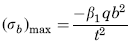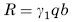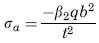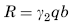Related Resources: calculators

Flat Rectangular Plate, Two Edges Fixed, Two Edges Free Uniform Loading over Plate from z = 0 to z = (2/3) b Equation and Calculator.

Per. Roarks Formulas for Stress and Strain for flat plates with straight boundaries and constant thickness

Flat Rectangular plate, Two Edges Fixed, Two Edges Free Uniform Loading over Plate from z = 0 to z = (2/3) b.

 Rectangular plate; Two Edges Fixed Two Edges FreeUniform Loading over plate from z = 0 to z = (2/3) bAt x = 0, z = 0
Stress and Reaction ForceReaction ForceStress and Reaction Force at x = 0, z = 0.6b if a > b/2 or z = 0.4b if a ≤ b/2Reaction ForceWhere used:
E = Modulus of Elasticity (lbs/in2)
q = Total load or force to Plate (lbs/in2)
v = Poisson’s ratio (assumed to be 0.3)
t = plate thickness, (in)
a = plate length, (in)
b = plate width, (in)
σb = stress, (lbs/in2)
R = Reaction Force (lbs/in)
β1,2 = Constant From Table A
γ1,2 = Constant From Table A

Table A

 a/b 0.125 0.25 0.375 0.5 0.75 1 β1 0.05 0.173 0.297 0.465 0.758 0.963 β2 0.044 0.143 0.23 0.286 0.396 0.435 γ1 0.311 0.543 0.563 0.654 0.741 0.748 γ2 0.126 0.249 0.335 0.377 0.384 0.393

Reference:

Roarks Formulas for Stress and Strain, 7th Edition